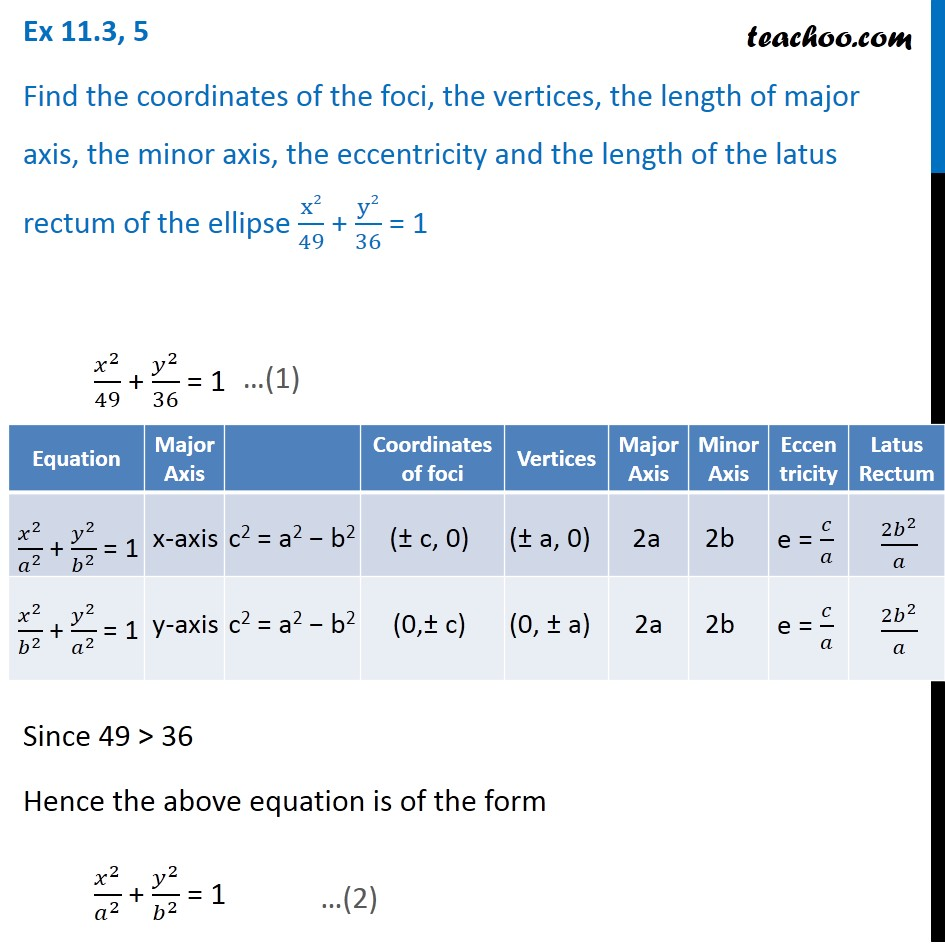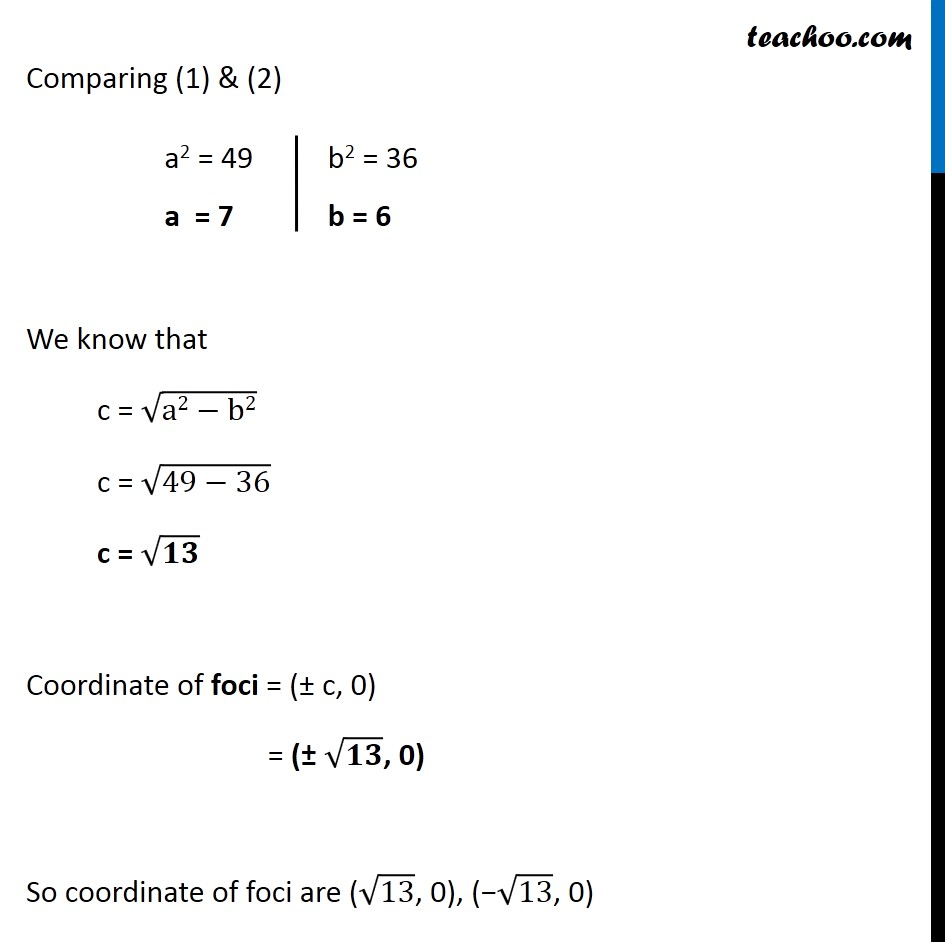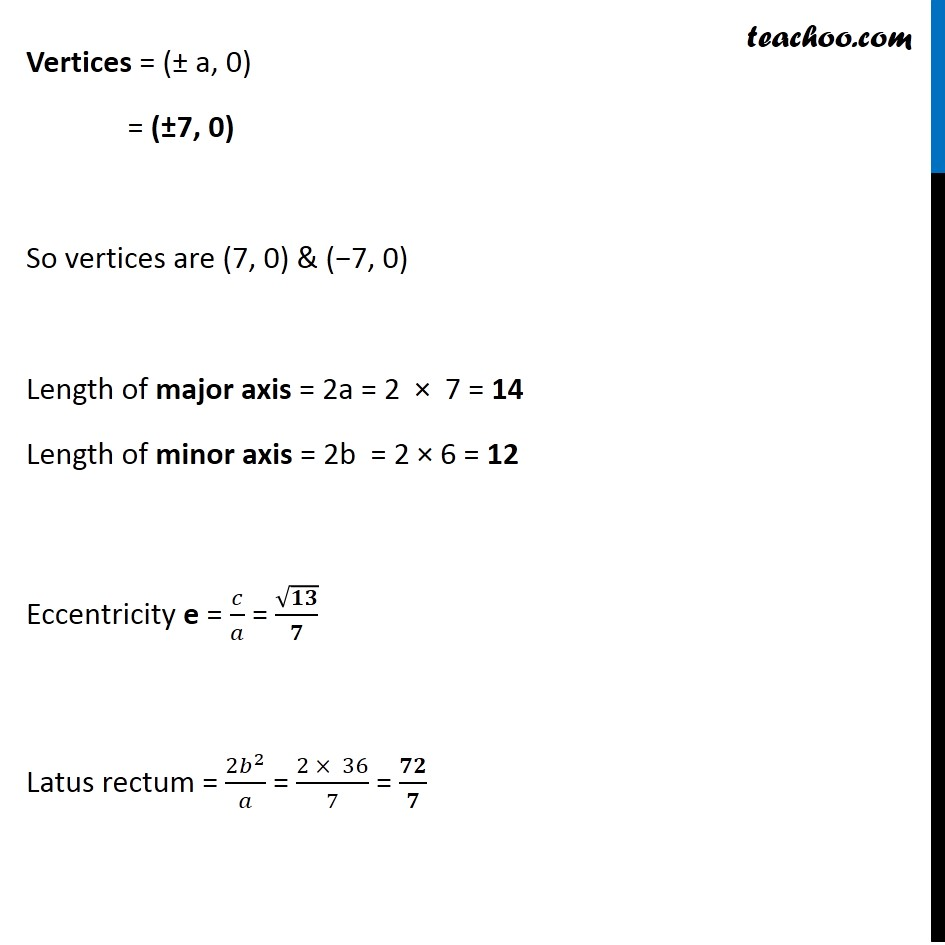Ellipse - Defination

Chapter 10 Class 11 Conic Sections
Concept wiseLearn in your speed, with individual attention - Teachoo Maths 1-on-1 Class

### Transcript

Ex 10.3, 5 Find the coordinates of the foci, the vertices, the length of major axis, the minor axis, the eccentricity and the length of the latus rectum of the ellipse x2/49 + y2/36 = 1 𝑥^2/49 + 𝑦^2/36 = 1 Since 49 > 36 Hence the above equation is of the form 𝑥^2/𝑎^2 + 𝑦^2/𝑏^2 = 1 Comparing (1) & (2) We know that c = √(a2−b2) c = √(49−36) c = √𝟏𝟑 Coordinate of foci = (± c, 0) = (± √𝟏𝟑, 0) So coordinate of foci are (√13, 0), (−√13, 0) Vertices = (± a, 0) = (±7, 0) So vertices are (7, 0) & (−7, 0) Length of major axis = 2a = 2 × 7 = 14 Length of minor axis = 2b = 2 × 6 = 12 Eccentricity e = 𝑐/𝑎 = √𝟏𝟑/𝟕 Latus rectum = (2𝑏^2)/𝑎 = (2 × 36)/7 = 𝟕𝟐/𝟕